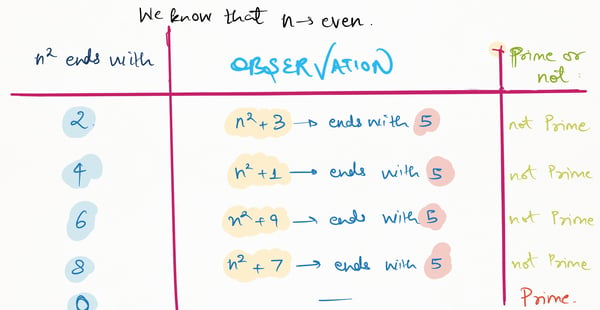Related Tags

project euler
python
communitycreator

# How to solve Project Euler 146: Investigating a prime pattern

Armaan Nougai

### Problem statement

10 is the least positive number for which the following are all consecutive primes:

  1) n^2+1
2) n^2+3
3) n^2+7
4) n^2+9
5) n^2+13
6) n^2+27


The sum of all such integers below 1 million is 1242490.

What is the sum of all such integers under one hundred fifty million?

### Question analysis

1. Our main goal here is to find the sum of such n under a hundred and fifty million, for which n^2+1, n^2+3, n^2+7, n^2+9, n^2+13, and n^2+27 are consecutive primes.
2. For the reason that the constrainta hundred and fifty million may be huge, we must lessen the scope of n.
3. After decreasing the scope of n, we will follow a quicker probable prime determining algorithm. Here, we can use the Miller-Rabin test.

### Reducing the scope of n

1. For n^2+1 to be prime, n^2 needs to be even. Subsequently, n must be even.

2. One important fact to consider here is that 5 is the only prime number that ends with 5. This means that any number that ends with 5 cannot be prime, besides 5 itself.So, from the above table, we can say that for n^2+1, n^2+3, n^2+7, n^2+13, and n^2+27 to be prime for a specific n, n^2 has to end with 0.

This means n has to be the multiple of 10. It also means that n can’t be a multiple of 3, 7, or 13.

### Primality test

To check if a number is possibly prime or not, we can use the Miller-Rabin primality test.

The Miller-Rabin primality test can only decide if a number is a potential prime, and is sufficient for this problem.

It is primarily based on the fact that:

If \$x^2 = (y^2)%N and x != y%N**,
then **N is composite.

### Python implementation

import random

def func_power(v, c, p):
res = 1

v = v % p
while (c > 0):
if (c & 1):
res = (res * v) % p

c = c>>1
v = (v * v) % p

return res

def miller_test(d, n):
a = 2 + random.randint(1, n - 4)
v = func_power(a, d, n)

if (v == 1 or v == n - 1):
return True

while (d != n - 1):
v = (v * v) % n
d *= 2;

if (v == 1):
return False
if (v == n - 1):
return True
return False

def prime(n, k=4):
if (n <= 1 or n == 4):
return False
if (n <= 3):
return True

d = n - 1
while (d % 2 == 0):
d //= 2

for i in range(k):
if (miller_test(d, n) == False):
return False

return True

sum1=0
for j in range(10,150000000,10):
if (j%3==0 or j%7==0 or j%13==0 )== True:
continue
t=j**2
sum1 += j if prime(t+1) and prime(t+3) and prime(t+7) and prime(t+9) and not prime(t+11) and prime(t+13) and not prime(t+17) and not prime(t+19) and not prime(t+21) and not prime(t+23) and prime(t+27) else 0

print(sum1)

RELATED TAGS

project euler
python
communitycreator

CONTRIBUTOR

Armaan Nougai
RELATED COURSES

View all Courses

Keep Exploring

Learn in-demand tech skills in half the time